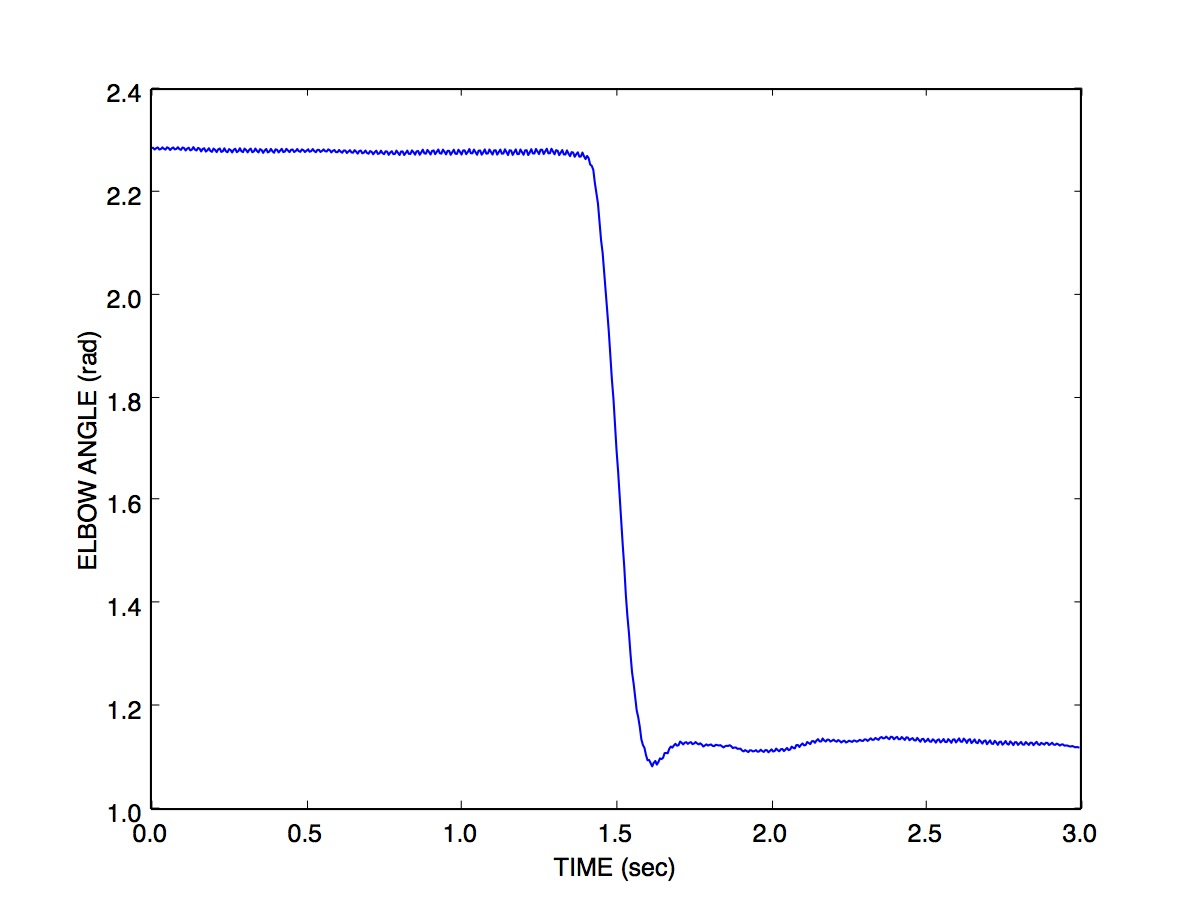# Exercise 32

## Scoring Kinematics

The file e31data.txt contains Optotrak measurements of a single-joint elbow rotation over time. The angle between the upper and lower arm (in radians) was sampled at 200 Hz. Here is a plot of the data file:Answer the following questions about the data. Use whatever signal processing techniques you wish, to answer each question. Explain the signal processing steps you took, and why.

### Position

Determine the angle (in degrees) that the elbow rotated over the course of the arm movement.

### Velocity

Determine the peak velocity, in radians per second, of the elbow movement. Hint: use the `gradient()` function to compute the central difference so you don't introduce a time lag and so the derivative has the same number of elements as the position array.

### Acceleration

Determine the peak positive and peak negative accelerations, in radians per second per second.

Paul Gribble | fall 2014